# Loci and Construction

GCSEKS3Level 4-5AQAEdexcelOCR

## Loci and Construction – The 4 Rules

In GCSE maths, there are 4 ruler and compass constructions you must to know.

1. Locus of all points around a point or line.
2. Perpendicular bisector.
3. Perpendicular line from a point.
4. Bisecting an angle.

## Loci

A locus (loci is the plural) is a collection of points which share a property.

E.g.  The circumference of a circle is the locus of all points in 2D that are the same distance from a particular point – the centre.

Example: Draw the locus of all the points $1$cm from line $AB$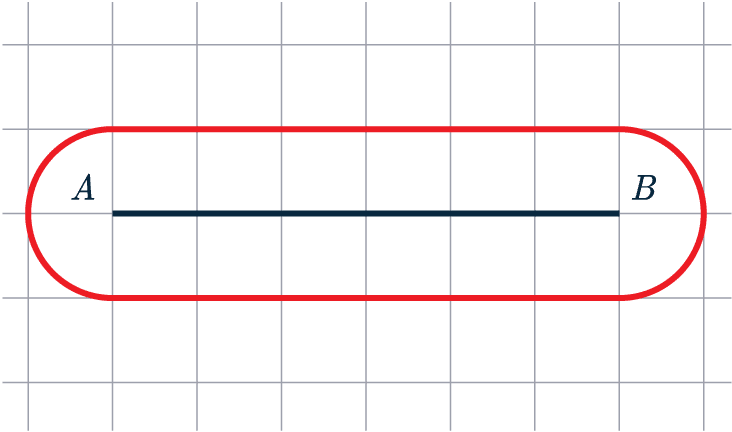– First we set the pair of compasses to $1$ cm, and draw a half circle around point $A$

– Next keeping the pair of compasses the same size, repeat for point $B$

– Finally, using a ruler join up the two circles.

All these steps are shown in the diagram in red.

We can see that every point on the red line is exactly $1$ cm away from the line $AB$.

Level 4-5GCSEKS3AQAEdexcelOCR

## Constructing a Perpendicular Bisector

The second construction is a perpendicular bisector of a line.

Example: Bisect line $AB$ shown below.

(or it could be asked as, draw a line equidistant from points $A$ and $B$, the method will be exactly the same.)

To do this you must do the following:

– Set your pair of compasses to a fixed length apart (Must be greater than half the line).

– Put your pair of compasses on point $A$ and draw an arc (blue).

– With your pair of compasses at the same length, repeat the previous step for the other end of the line (also blue).

– Then, draw a line which passes through the two crossing points. This line (red) is the perpendicular bisector.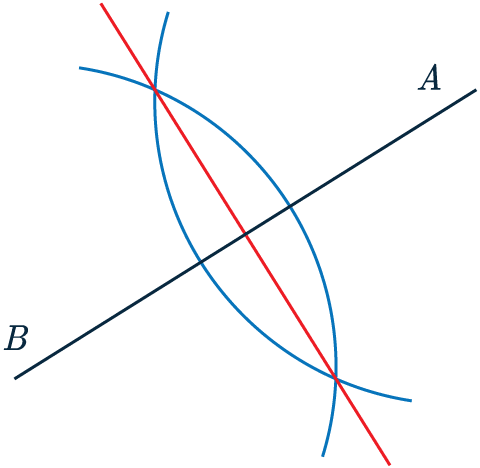Level 4-5GCSEKS3AQAEdexcelOCR

## Perpendicular Line From a Point

The third construction is a perpendicular line from a point.

In the diagram, we have been given a line segment and a point (both black).

Example: Construct a perpendicular line from point $C$ to line $AB$.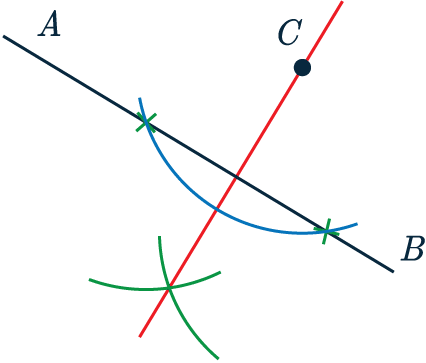– Place your pair of compasses on the point $C$ (pair of compasses must be set greater than half the length of the line) and draw an arc of a circle that passes through the line twice (blue).

– Place your pair of compasses on the crossing point (green cross) and draw a small arc on the opposite side of the line to where the point is (green).

– With your pair of compasses at the same length, repeat previous step for the other crossing point (other green cross).

– Draw a line that passes through the original point and the point where the last two arcs cross.

This line (red) is the perpendicular line from a point.

Level 4-5GCSEKS3AQAEdexcelOCR

## Bisecting an Angle

The fourth construction is an angle bisector. This allows us to split a given angle perfectly in half.

Example: Construct a line, equidistant from line $AB$ and $BC$.

In the diagram, we have been given two lines (black) and an angle between them. To bisect it, we do the following: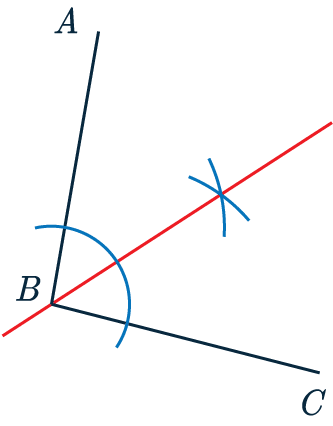– Place your pair of compasses on the corner where the two lines meet and draw an arc (blue) that passes through both lines.

– Place your pair of compasses on the two crossing points of the blue arch and draw a small arc, shown in blue.

– With your pair of compasses at the same length, repeat previous step, from the other crossing point.

– Draw a line (red) passing through the corner where the lines meet and the point where the two blue arcs cross. This is the angle bisector.

Level 4-5GCSEKS3AQAEdexcelOCR

## Example: Loci and Construction

Points $A$ and $B$ are on a straight line.

Shade the locus of points that are closer to point $A$ than point $B$ but less than $4$cm from point $B$.

[3 marks]

Firstly, we need to find the points that are “closer to point $A$ than point $B$. This means all the points that are to the left of the line which fall halfway between $A$ and $B$. This means we need to draw a perpendicular bisector.

Following the steps, detailed above, we get the perpendicular bisector of line $AB$, shown by the red line.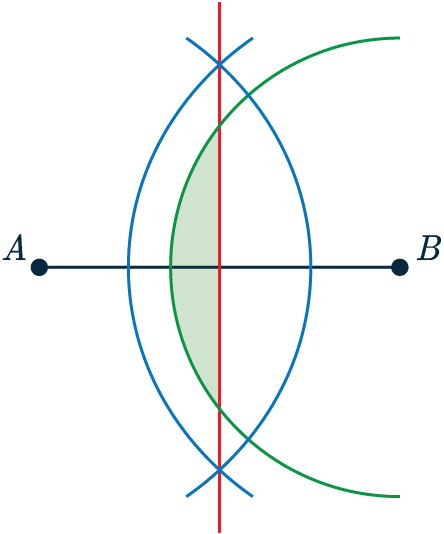Next, we need to find the set of points that are less than $4$ cm from $B$, for this we use the locus of points around a point.

We set out our pair of compasses to a length of $4$ cm, then placing the needle on $B$ we draw our circle.

Finally, we shade all the points beyond the orange line but closer then $4$ cm from $B$.

This can be seen in green on the diagram.

Level 4-5GCSEKS3AQAEdexcelOCR

## Loci and Construction Example Questions

So, we place the compass at B and draw an arc that passes through both lines.

Then, placing the compass at each of the points where the first arc crossed, we draw two small arcs which lie in between lines AB and BC.

The line which passes through the corner at B and the point where the latter two arcs cross is the angle bisector, which is the locus of points which are equidistant from AB and BC. The correct picture should look like the one below (construction lines in blue, locus in red):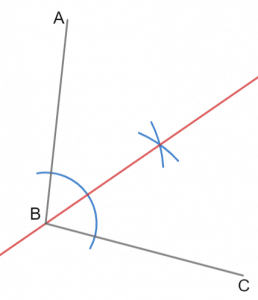Gold Standard Education

Firstly, the locus of points which are 1cm directly above the line is another line, 1cm away from, and parallel to, this line. Same goes for the locus directly below the line.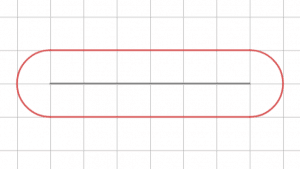Gold Standard Education

So, for the fountain to be at least 3m away from his house along CD, we need to only consider the area to the left of the straight line which is parallel to CD and 3cm away from it.

Then, the locus of points which are 1.5m away from the tree at E will be a circle of radius 1.5cm – for the fountain to be at least 1.5m away, it must be outside this circle.

So, the locus of points where he could place the fountain is to the left of the (blue) line 3m away from the house, and outside the (green) circle which is 1.5m away from the tree. The correct region is shaded red on the picture below.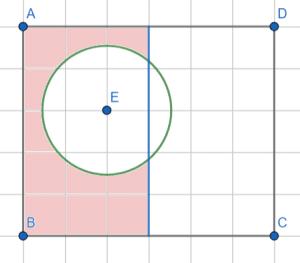Gold Standard Education

The bisector of an angle is a line segment which divides the angle into two equal parts.Gold Standard Education

Construction of a line perpendicular to AB passing through point P as shown: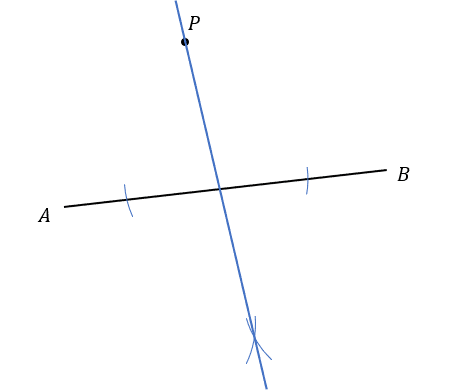Gold Standard Education

## Loci and Construction Worksheet and Example Questions

### (NEW) Loci & Constructions Exam Style Questions - MME

Level 4-5GCSENewOfficial MME

## Loci and Construction Drill Questions

Level 4-5GCSE

### Loci And Construction 2 - Drill Questions

Level 4-5GCSEGold Standard Education

£19.99 /month. Cancel anytime